# Designing an AND Gate using Transistors

Published  October 14, 2019   1
J
Author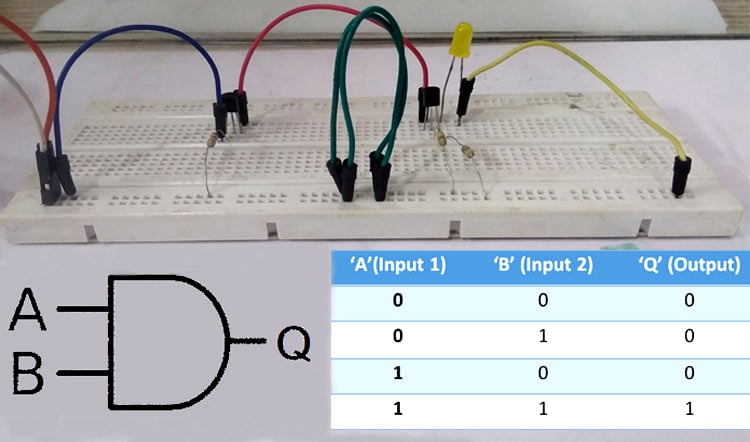As many of us know an Integrated Circuit or IC is a combination of many small circuits in a small package which together performs a comman task. Like an Operational Amplifier or 555 Timer IC is built by combination of many Transistors, Flip-Flops, Logic Gates and other combinational digital circuits. Similarly a Flip-Flop can be built by using a combination of Logic Gates and the Logic Gates itself can be built by using a few Transistors.

Logic Gates are the basics of a many digital electronic circuit. From the basic Flip-Flops to Microcontrollers Logic gates form the underlying principle on how bits are stored and processed. They state the relation between every input and output of a system using an Arthmetic logic. There are many different types of logic gates and each of them has a different logic which be used for different purposes. But the focus of this article will be on the AND Gate because later we would be building an AND Gate using a BJT transistor circuit. Exciting right? Lets get started.

### AND Logic Gate

AND logic gate is a D-shaped logic gate with two inputs and one single output, where the D shape in between the input and output is the logic circuit. The relation between input and output values can be explained using the AND Gate Truth Table shown below.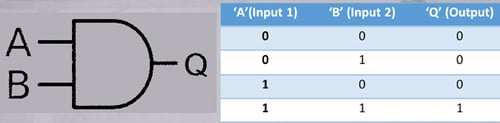The equations output can be easily explained using the AND Gate Boolean Equation, which is Q = A x B or Q = AB. Hence, for an AND Gate the output is HIGH only when both the inputs are HIGH.

### Transistor

A transistor is a semiconductor device with three terminals that can be connected to an external circuit. The device can be used as a switch and also as an amplifier to change the values or control the passing of an electrical signal.

For building an AND logic gate using a transistor we would be using BJT transistors which can be further classified into two types: PNP and NPNBipolar Junction Transistors. The circuit symbol for each of them can be seen below.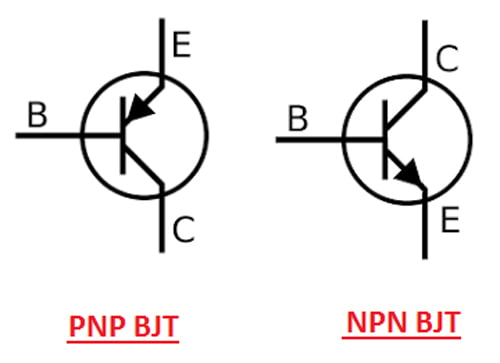This article will explain to you, how to build AND Gate circuit using transistor. The logic of an AND gate is explained above already and to build an AND gate using a transistor we will follow the same truth table shown above.

### Circuit Diagram and Components Required

The list of components required to build an AND gate using an NPN transistor are listed as follows:

1. Two NPN transistors. (You can also use PNP transistor if available)
2. Two 10KΩ resistors & one 4-5KΩ resistor.
3. One LED (Light Emitting Diode) to check the output.
5. A +5V Power supply.
6. Two PUSH buttons.
7. Connecting Wires.

The circuit represents both the inputs A & B for the AND gate and Output, Q which also has a +5V supply to the collector of the first transistor which is connected in series to the second transistor and an LED is connected to the emitter terminal of the second transistor. The inputs A & B are connected to the base terminal of Transistor 1 and Transistor 2, respectively and the output Q goes to the positive terminal LED. The below diagram represents the above-explained circuit to build an AND gate using NPN Transistor.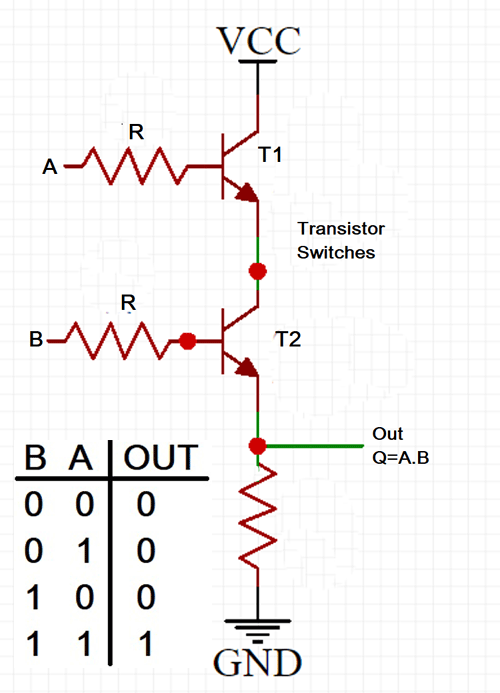The Transistors used in this tutorial are BC547 NPN Transistor and were added with all the above-mentioned components in the circuit, as shown below.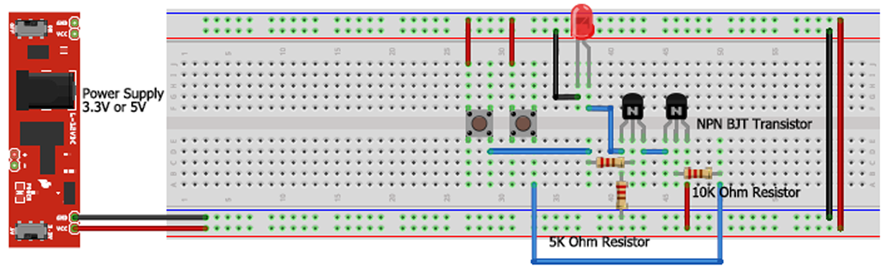If you do not have the push buttons with you, you can also use wires as a switch by adding or removing them whenever required (instead of pressing the swtich). The same could be seen in the video where I would use the wires as a switch connected to the base terminal for both the transistors.

The same circuit when built using the above mentioned hardware components, the circuit would look something like in the image below.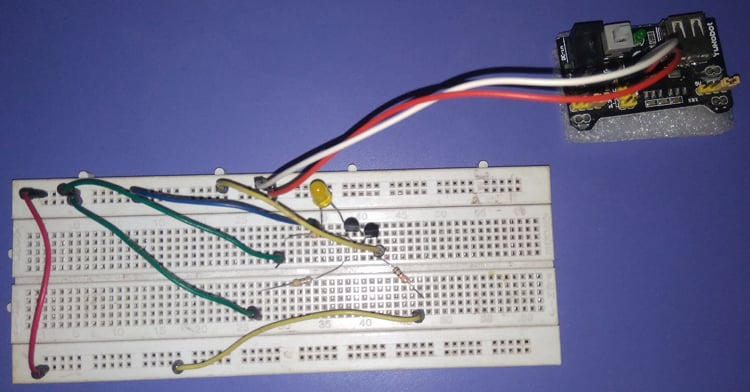### Working of And Gate using Transistor

Here we will be using the transistor as a switch and so, when a voltage is applied through a Collector terminal of the NPN transistor, the voltage reaches the Emitter Junction only when the Base Junction is having a voltage supply between 0V and Collector Voltage.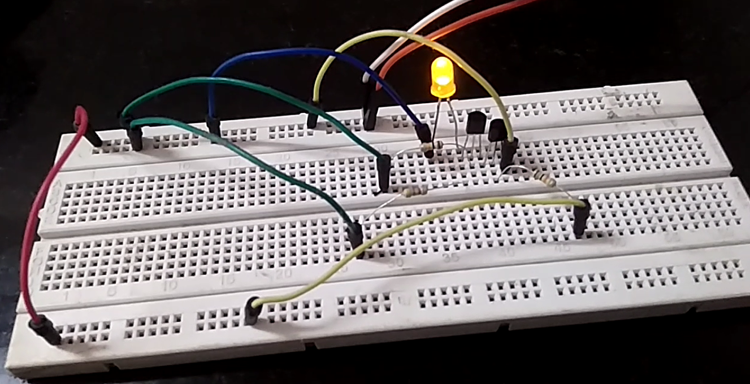Similarly, the circuit above would make the LED glow i.e. the output is 1 (High) only when both the inputs are 1 (High) i.e. when there is a voltage supply at the base terminal of both the transistors. Meaning, there will be a straight line current path from VCC (+5V power supply) to the LED and further to the ground. Rest in all the cases, the output will be 0 (Low) and the LED will be OFF. These all can be explained in more detail by understanding each case one by one.

Case 1: When both inputs are zero – A = 0 & B = 0.

When both the inputs A & B are 0, you need not press any of the pushbuttons in this case. If you are not using the push buttons then remove the wires connected with, the pushbuttons and the base terminal of both the transistors. So, we got both inputs A & B as 0 and now we need to check for the output, which also should be 0 according to the AND gate truth table.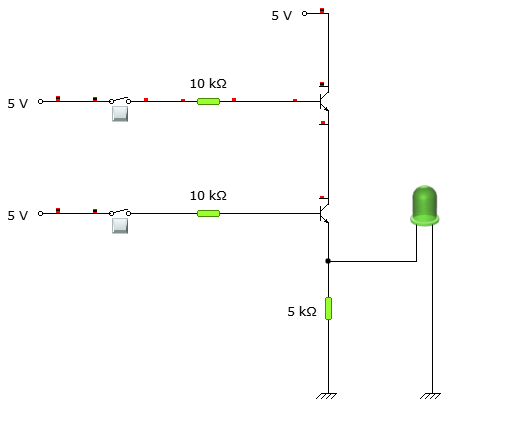Now, when a voltage is supplied through the collector terminal of Transistor 1, the emitter doesn’t receive any input because the base terminal value is 0. Similarly, the emitter of transistor 1 which is connected to the collector of Transistor 2, supplies no current or voltage and also the base terminal value of transistor 2 is 0. So, the 2nd transistor’s emitter outputs the value 0 and as a result, the LED would be OFF.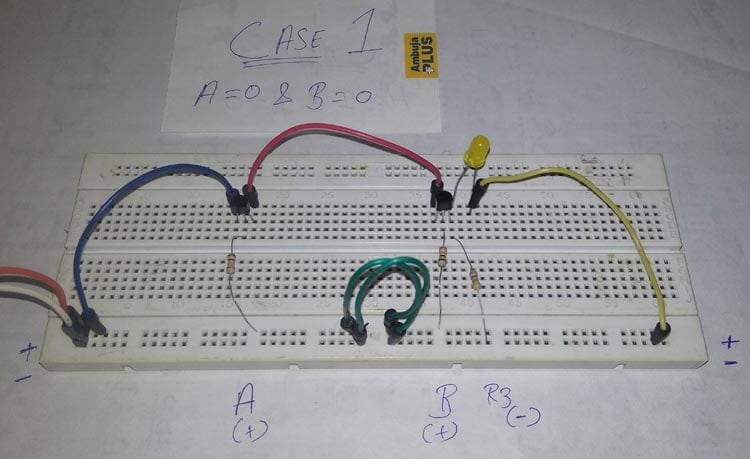Case 2: When the inputs are – A = 0 & B = 1.

In the second case, when the inputs are A = 0 & B = 1, the circuit has first input as 0 (Low) and the second input as 1 (High) to the base of transistor 1 & 2, respectively. Now, when a 5V supply is passed to the collector of the first transistor, then there is no change in the phase shift of transistor since that the base terminal has 0 input. Which passes 0 value to the emitter and the emitter of first transistor is connected to the collector of the second transistor in series, so 0 value goes into the collector of second transistor.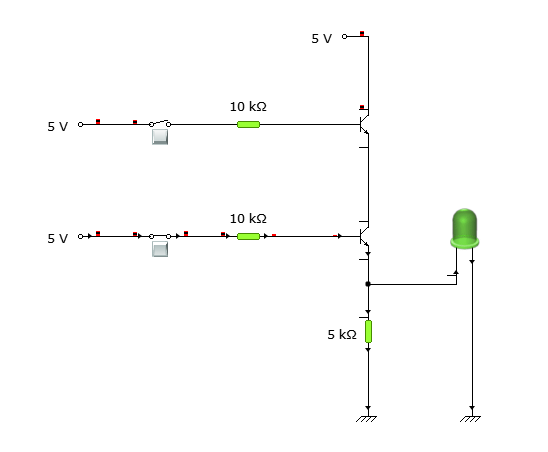Now, the second transistor has a high value in the base, so it would allow the same value received in the collector to pass to the emitter. But since the value is 0 in the collector terminal of the second transistor, that’s why the emitter will also be 0 and the LED connected to the emitter would not glow.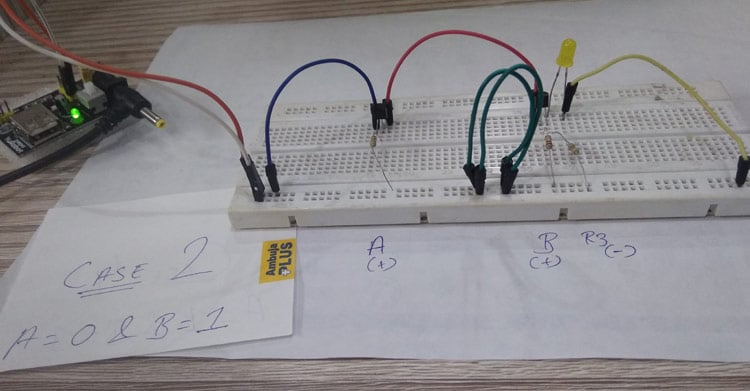Case 3: When the inputs are – A = 1 & B = 0.

Here, the input is 1 (high) for the first transistor base and low for the second transistor base. So, the current path will start from 5V power supply to the collector of the second transistor passing through the collector and emitter of the first transistor since the base terminal value is high for the first transistor.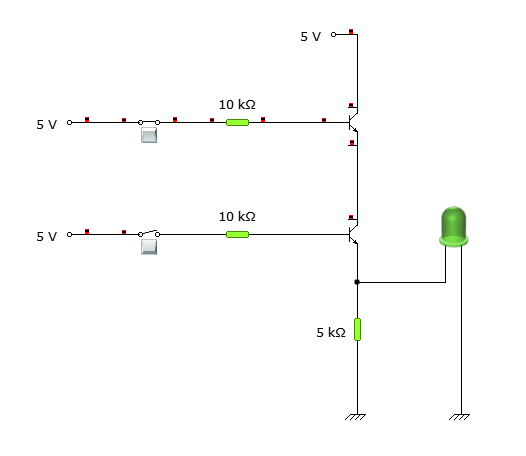But in the second transistor, the base terminal value is 0 and so, no current passes from collector to the emitter of the second transistor and as a result, the led would still be OFF only.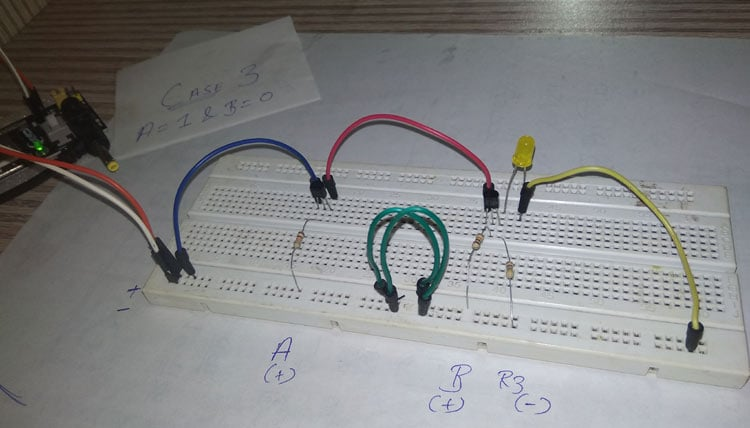Case 4: When both inputs are one – A = 1 & B = 1.

The last case and here both the inputs are supposed to be high which are connected to the base terminals of both the transistors. This means whenever a current or voltage passes through the collector of both the transistors, the base reaches its saturation and the transistor conducts.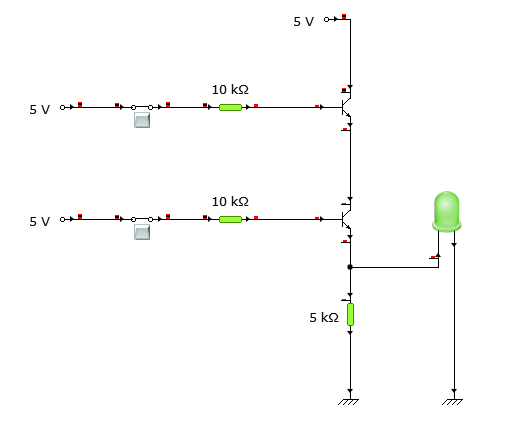Practically explaining, when a +5V supply is provided to the collector terminal of transistor 1 and also the base terminal is saturated then, the emitter terminal would receive a high output since the transistor is forward biased. This high output at the emitter goes directly to the collector of 2nd transistor through a series connection. Now, similarly at the second transistor, the input at the collector is high and in this case, the base terminal is also high, meaning the second transistor is also in a saturated state and the high input would pass from the collector to the emitter. This high output at the emitter goes to the LED which turns the LED ON.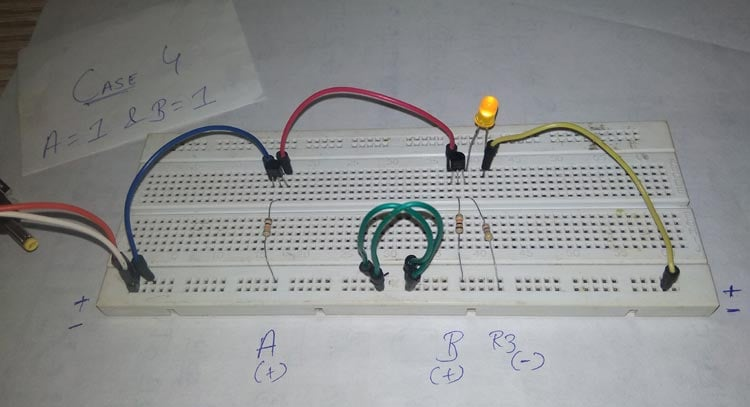Hence, all four cases have the same inputs and outputs as the actual AND logic gate. Thus, we have built an AND Logic gate using a Transistor. Hope you understood the tutorial and enjoyed learning something new. The complete working of the set-up can be found in the video below. In our next tutorial we will also learn how to build OR gate using transistor and NOT gate using Transistor. If you have any questions leave them in the comment section below or use our forums for other technical questions.

Video